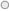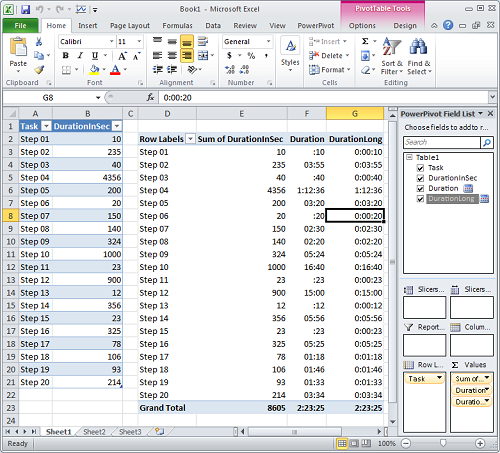# Q: I have integer amount that represents duration in seconds. How can show this amount in format "h:mm:ss"?Q: I have integer amount that represents duration in seconds. How can show this amount in format "h:mm:ss"?

A: Create new calculated DAX measure "Duration". Formula for this measure will be:

=IF(SUM(Table1[DurationInSec])<60*60,"",FLOOR(SUM(Table1[DurationInSec])/60/60,1) & ":")
& IF(SUM(Table1[DurationInSec])<60,"", RIGHT("0" & FLOOR(MOD(SUM(Table1[DurationInSec]),3600)/60,1),2))
& ":" & RIGHT("0" & MOD(SUM(Table1[DurationInSec]),60),2)

Now when we use calculate DAX measure "Duration" in our reports we will see result in a form "h:mm:ss".

Note: above formula generates short form of "h:mm:ss" were "h:mm" part is optional:

• when duration is less than 1 hour then we do not display "h:" part.
• when duration is less than 1 minutes, then we do not display "mm:" part.

If you always want to see hour and minute part, you should use slightly adjusted DAX formula (measure "Duration Long"):

=IF(SUM(Table1[DurationInSec])<60*60,"0:", FLOOR(SUM(Table1[DurationInSec])/60/60,1) & ":")
& IF(SUM(Table1[DurationInSec])<60,"00", RIGHT("0" & FLOOR(MOD(SUM(Table1[DurationInSec]),3600)/60,1),2))
& ":" & RIGHT("0" & MOD(SUM(Table1[DurationInSec]),60),2)

Screenshot:Tags: dax, excel, time

2007-2015 VidasSoft Systems Inc.